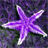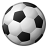# How to display the centroid of a circle

1671
7
10-31-2013 11:30 AMNew Contributor II
My company just switched us from MapInfo to Arcmap.

Is there a way to easily display the centroid of a circle in ArcMap? Or do I have to somehow calculate the centroid using some tool or function?

In MapInfo, we just checked "show centroids" in the layer control box.
Tags (2)
1 Solution

Accepted SolutionsbyMVP Honored Contributor
You can add two double fields for the X and Y coordinate to the polygons and use the geometry calculator to calculate the centroid coordinate values into them.  You could alternatively calculate the coordinates with the Field Calculator and the Python expression: !Shape.Centroid.X! and !Shape.Centroid.Y!.  Then export from the table view to create a table that contains the coordinate.  Then use the Make XY Event Layer to make a layer showing the points based on the coordinate fields you calculated.
7 Repliesby
Frequent Contributor
Mike,

You can use this tool to create a centroid layer.

TimNew Contributor II
Mike,

You can use this tool to create a centroid layer.

Tim

Thanks, I'm sure that would work, however, I get a "tool not licensed" dialog box. I guess I could try licensing the tool...
Any other ideas?by
Regular Contributor III
It's not the tool that's licensed, precisely, it's the version of ArcMap you're using. The tool Tim mentions requires an ArcInfo license, the most advanced of the three.

You might try the free version of ET GeoWizards (or buy the full product). The free version has tools that allow you to calculate the x & y of centroids.byMVP Honored Contributor
You can add two double fields for the X and Y coordinate to the polygons and use the geometry calculator to calculate the centroid coordinate values into them.  You could alternatively calculate the coordinates with the Field Calculator and the Python expression: !Shape.Centroid.X! and !Shape.Centroid.Y!.  Then export from the table view to create a table that contains the coordinate.  Then use the Make XY Event Layer to make a layer showing the points based on the coordinate fields you calculated.by
Regular Contributor III
Better idea than mine, Richard, I forgot you could do that in Arc, don't need ET to calculate that.New Contributor II
You can add two double fields for the X and Y coordinate to the polygons and use the geometry calculator to calculate the centroid coordinate values into them.  You could alternatively calculate the coordinates with the Field Calculator and the Python expression: !Shape.Centroid.X! and !Shape.Centroid.Y!.  Then export from the table view to create a table that contains the coordinate.  Then use the Make XY Event Layer to make a layer showing the points based on the coordinate fields you calculated.

Thanks! That works great! I also found an article outlining what you just said about the geometry calculator here.New Contributor II
It's not the tool that's licensed, precisely, it's the version of ArcMap you're using. The tool Tim mentions requires an ArcInfo license, the most advanced of the three.

You might try the free version of ET GeoWizards (or buy the full product). The free version has tools that allow you to calculate the x & y of centroids.

I also tried this and got it to work. Under the "Convert" tab, I used the "polyline to point" tool.

Thanks!